# calculus of variations

Also found in: Dictionary, Thesaurus, Acronyms, Wikipedia.

## calculus of variations,

branch of mathematicsmathematics,
deductive study of numbers, geometry, and various abstract constructs, or structures; the latter often "abstract" the features common to several models derived from the empirical, or applied, sciences, although many emerge from purely mathematical or logical
concerned with finding maximum or minimum conditions for a relationship between two or more variables that depends not only on the variables themselves, as in the ordinary calculuscalculus,
branch of mathematics that studies continuously changing quantities. The calculus is characterized by the use of infinite processes, involving passage to a limit—the notion of tending toward, or approaching, an ultimate value.
, but also on an additional arbitrary relation, or constraint, between them. For example, the problem of finding the closed plane curve of given length that will enclose the greatest area is a type of isoperimetric (equal-perimeter) problem that can be treated by the methods of the variational calculus; the solution to this special case is the circle. Another famous problem is the brachistochrone problem, that of finding the curve along which an object will slide to a point not directly below it in the shortest time; the solution is a cycloid curve (a curve traced out by a fixed point on the circumference of a circle as the circle rolls along a straight line). In general, problems in the calculus of variations involve solving the definite integral (single or multiple) of a function of one or more independent variables, x1, x2, … , one or more dependent variables, y1, y2, … , and derivatives of these, the object being to determine the dependent variables as functions of the independent variables such that the integral will be a maximum or minimum. The calculus of variations was founded at the end of the 17th cent. and was developed by Jakob and Johann Bernoulli, Isaac Newton, G. W. Leibniz, Leonhard Euler, J. L. Lagrange, and others.

## Calculus of Variations

a mathematical discipline devoted to finding extremal (largest or smallest) values of functionals—variable quantities that depend on the choice of one or several functions. The calculus of variations is a natural development of that part of mathematical analysis that is devoted to the problem of finding the extrema of functions. The origin and development of the calculus of variations is closely connected with problems in mechanics, physics, and other sciences.

One of the first problems of the calculus of variations was the well-known brachistochrone problem (J. Bernoulli, 1696). This problem involves determining the shape of the curve that lies in a vertical plane and along which a heavy material point, moving under the influence of gravity only and having no initial velocity, passes from an upper position A to a lower position B in the shortest time. The problem reduces to finding the function y(x) that yields the minimum of the functional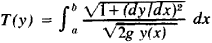where a and b are the abscissas of points A and B.

Another such “historic” problem is that of finding the path along which light traveling from a light source (point A) toward a certain point B is propagated in a medium with a variable optical density (that is, in a medium where the propagation velocity v is a function of the coordinates). The so-called Fermat principle can be used for the solution of this problem. According to this principle, of all the curves connecting points A and B, the light ray is propagated along the one for which light proceeds from A to B in the shortest time. In the simplest case, when light is propagated in a plane, the problem reduces to finding the curve y(x) that furnishes the minimum of the functional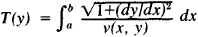From unrelated, similar-type problems, the calculus of variations began to develop gradually in the 18th century. But even after the calculus of variations became an independent discipline, it continued to be connected with various problems in mechanics and physics. Throughout the second half of the 18th century and all of the 19th century, intensive efforts were made to construct a foundation of mechanics based on certain general variational principles. From the second half of the 19th century on, various variational principles in continuum mechanics, and later in quantum mechanics, electrodynamics, and other fields, began to be developed. Variational principles also arise in media in which energy dissipation occurs. Research in all similar fields continues to serve as a basis for the formation of new problems in the calculus of variations and as a field of application of its methods. However, new classes of problems appeared with time, which far extended the traditional boundaries of the discipline and transformed the calculus of variations into one of the broadest branches of modern mathematics, including, on the one hand, the most abstract problems pertaining to topology and functional analysis, and on the other, diverse computational methods for solving technical and economic problems.

Direct methods. The calculus of variations developed as an independent scientific discipline in the 18th century, chiefly owing to the work of I. Euler.

The simplest problem of the calculus of variations is the problem of finding the function x(t) that furnishes an extremum of the functional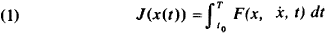where F is a continuous and differentiable function of its own arguments. In this case, the function x(t) must satisfy the following conditions: (a) it must be stepwise differentiable and (b) for t = t0 and t = T, it must take on the values

(2) x(t0) = x0x(T) = xT

The two problems considered at the beginning of this article are special cases of the simplest problem of the calculus of variations.

The first variational problems were problems in mechanics. They were posed in the 18th century, and following the traditions of the time, the first question that had to be answered was that of a practical method of finding a function x(t) that would realize the minimum of functional (1).

Euler developed a numerical method of solving variational problems, which became known as the Euler method of broken arcs. This method was the first of a large class of so-called direct methods; they are all based on the reduction of the problem of finding an extremum of a functional to the problem of finding the extremum of a function of many variables. Since, to obtain a highly accurate solution, the problem must be reduced to a problem of finding the extremum of a function having many variables, it becomes too complex for manual calculation. Therefore, the direct methods were for a long time outside the mainstream of the efforts of the mathematicians who were working with the calculus of variations.

Interest in the direct methods intensified in the 20th century. To begin with, new methods of reduction to a problem of finding the extremum of a function of a finite number of variables were proposed. Let us illustrate these ideas with a simple example. Let us consider again the problem of finding the minimum of functional (1) with the additional condition

(3) x(t0) = x(T) = 0

and let us search for a solution of the form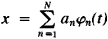where φn(t) is some system of functions satisfying conditions of the type of relation (3). Then the functional J(x) becomes a function of the coefficients at:

J = J(a1,…,aS)

and the problem is reduced to that of finding the minimum of this function of N variables. Under known conditions imposed on the system of functions {φn}, the solution to this problem converges to the solution to problem (1) as N → ∞.

Another cause of the increasing interest in direct methods was the systematic investigation of finite-difference methods in problems of mathematical physics, which began in the 1920’s. The use of computers is gradually converting the direct methods into the primary instrument for solving variational problems.

Method of variations. A second approach in solving variational problems was the investigation of the necessary and sufficient conditions that must be satisfied by a function x(t), which is the extremum of functional J(x). Its emergence is also connected with Euler. Let us assume that one or another method is used to construct the function x(t). How do we verify whether this function is the solution of the problem? The first variant of the answer to this question was given by Euler in 1744. In the formulation of this answer given below, the concept of variation (hence the designation calculus of variations) introduced in the 1860’s by J. Lagrange is used; this concept is a generalization of the concept of the differential for the case of functionals.

Let x(t) be a function satisfying condition (2) and h(t) an arbitrary smooth function satisfying the condition h(t0)= h(T) = 0. Then the quantity

J(x + ∊h) =J*(∊)

where ∊ is an arbitrary real number, will be a function of ∊. The variation δJ of the functional J is the derivative (dJ*/d∊)=0

For the simplest problem of the calculus of variations,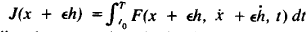Expanding the expression obtained in a series in powers of ∊, we obtain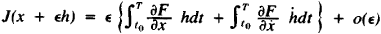where o(∊) represents higher order terms. Because h(t0) = h(T) = 0, then, carrying out integration by parts in the second integral, we find thatNow, let x(t) realize an extremum. Then the function J*(∊) has an extremum at ∊ = 0. Therefore, the quantity δj must vanish. Hence, it follows that in order that the function x(t) furnish an extremum to the functional (1), it is necessary that it satisfy the equation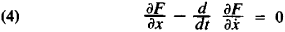called the Euler equation.

This is a second-order differential equation with respect to function x(t). The necessary condition δJ = 0 can be applied in a number of cases for the effective determination of the solution of the variational problem, since function x(t) must necessarily be the solution of the boundary value problem x(t0) = x0, X(T) = xT for equation (4). If this solution is found and it is unique, then the exact solution of the initial variational problem is also found. If the boundary value problem allows several solutions, then it is sufficient to calculate the value of the functional for each of the solutions of the boundary value problem and to select the one which corresponds to the extreme value of J(x). However, the indicated method has one essential shortcoming: there are no universal methods of solving boundary value problems for ordinary (nonlinear) differential equations.

By the second half of the 18th century, the range of problems being studied in the calculus of variations had broadened considerably. To begin with, the fundamental results pertaining to the simplest problem of the calculus of variations were carried over to the general case of integral functionals of the form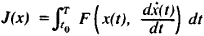where x(t) is a vector function of arbitrary dimension, and to functionals of still more general form.

Conditional extremum. Lagrange problem. At the end of the 18th century a number of problems were formulated for the conditional extremum. This term is used to describe the problem of finding a function x(t) that furnishes an extremum of the functional J(x) under some auxiliary conditions besides the conditions on the ends of the interval (t0, T). The simplest problem of similar form is the class of so-called isoperimetric problems. This class of problems, as the name suggests, involves the following: to find, among all the closed curves of a given perimeter, the one that confines the maximum area.

A considerably more complex problem is that in which the restrictions are in the form of differential equations. This problem is called the Lagrange problem; it acquired special significance in the middle of the 20th century in connection with the creation of the theory of optimal control. Consequently, its formulation is given below in the language of this theory, which was begun in the work of L. S. Pontriagin and his students.

Let x(t) and u(t) be vector functions of dimension n and m, respectively, while the function x(t), which is called a phase vector, satisfies, for t = t0 and t = T, the boundary conditions

(5) x(t0Є x(T)ЄЄT

where ∊0 and ∊T are certain sets. The simplest example of conditions of the type given in expressions (5) are conditions (2). Function x(t) and function u(t), which is called the control, are connected by the condition

(6) dx/dt = f(x, u, t)

where f is a differentiable vector function of its own arguments. The problem under consideration consists of determining functions x(t) and u(t), which furnish an extremum to the functional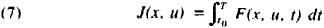Let us note that both the simplest problem of the calculus of variations and the isoperimetric problem are special cases of the Lagrange problem.

The Lagrange problem has vast value in applied mathematics. For example, let equation (6) describe the motion of some dynamic object, for example, a spacecraft. The control u is the thrust vector of its engine. The sets ∊0 and ∊T are two orbits of different radii. The functional (7) describes the fuel consumption in the execution of a maneuver. Consequently, the Lagrange problem applicable to the given situation can be formulated in the following manner: determine the law of variation of the thrust of the engine of a spacecraft that is effecting a transition from orbit ∊0 to orbit ∊T within a given time so that the fuel consumption for this maneuver be minimal.

An important role in the theory of similar problems is played by the Hamiltonian function

H(x, ψ u) = (f, ψ) - F

Here, ψ is a vector called the Lagrange multiplier (or momentum) and (f, ψ) denotes the scalar product of vectors f and ψ. The necessary condition in the Lagrange problem is formulated in the following manner: in order that the functions x˜(t) and ū(t) be the solution of the Lagrange problem, it is necessary that ū(t) be a stationary point of the Hamiltonian function H(x, ψ u)—that is, ∂H/∂u must equal 0 for u = ü—where ψ is a not identically equal to zero solution of the equation

(8) ∂ψ/∂t = – ∂H/∂x = ψx, ψ, u, t)

This theorem is of great value in applied mathematics, because it opens up certain possibilities for the practical calculation of vectors x(t) and u(t).

Development in the 19th century. The primary efforts of mathematicians in the 19th century were directed at the study of the conditions that were necessary or sufficient for the function x(t) to realize an extremum of functional J(x). The Euler equation was the first of such conditions; it is analogous to the necessary condition df/dx = 0, which is established in the theory of functions of a finite number of variables. However, there are also other conditions known in this theory. For example, in order that function f(x) have a minimum at the point x-, it is necessary that this point [h, (d2f/dx2)h] ≥ 0, no matter what arbitrary vector h is chosen. It is natural to pose the problem: to what extent are these results carried over to the case of functionals? In order to visualize the complexity arising here, let us note that function x˜(t) can achieve a minimum among the functions of one class and not yield a minimum among the functions of another class.

Similar problems served for a while as the source of the diverse and detailed investigations of A. Legendre, G. Jacobi, M. V. Ostrogradskii, W. Hamilton, K. Weier-strass, and many other mathematicians. These investigations not only enriched mathematical analysis but also played an important role in the formation of the ideas of analytical mechanics and exerted an important influence on the development of various branches of theoretical physics.

Development in the 20th century. In the 20th century a number of new directions were taken in the study of the calculus of variations, which were connected with the intensive development of technology and related problems in mathematics and computer technology. One of the principal trends was the treatment of nonclassical variational problems, which led to the discovery of L. S. Pontriagin’s principle of the maximum.

Let us again consider the Lagrange problem: determine the minimum of the functional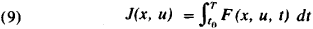with the conditions x˜= f(x, u); the phase vector x(t) must still satisfy certain boundary conditions.

In its classical formulation, the conditions of the Lagrange problem do not provide for any restrictions on the control u(t). In the above (see above: Conditional extremum. Lagrange problem) the close connection between the Lagrange problem and control problems was emphasized. In the example considered there, u(t) is the rocket engine’s thrust. This quantity conforms to the restriction that the engine’s thrust cannot exceed a certain value; the steering angle of the thrust vector is also restricted. In the given concrete example the component ui (i = 1, 2, 3) of the thrust vector is limited by the restrictionswhere a1- and ai+ are certain given numbers. Many similar examples can be cited.

Thus, many problems arise in technology that reduce to the Lagrange problem, but with additional restrictions of the type in relation (10), written in the form u ∊ Gu, where Gu is some set which, in particular, can be closed. Such problems have been designated as optimal control problems. In the Lagrange problem, the control u(t) can be eliminated using equation (8) and a system of equations can be obtained containing only the phase variable x and the Lagrange multiplier ψ. For the theory of optimal control, special apparatus has had to be developed. These investigations led to the discovery of Pontriagin’s principle of the maximum. It can be formulated in the form of the following theorem: in order that functions x¯(t) and ū(t) be the solution to an optimal control problem [so that they could furnish a minimum of the functional (9)], it is necessary that ü(t) furnish a maximum of the Hamiltonian functionwhere ψ is a Lagrange multiplier (momentum), which is a nonzero solution of the vector equation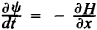The principle of the maximum permits the reduction of an optimal control problem to a boundary value problem for a system of ordinary differential equations of order 2n (n is the dimension of the phase vector). The principle of the maximum in this case also yields a stronger result than Lagrange’s theorem, since it requires that ū not be a stationary value of the Hamiltonian function H but that it furnish a maximum of H.

Another approach to the same problems of optimal control theory is also possible. Let s(x,t) be the value of functional (9) along the optimal solution. Then, in order that function u(t) be an optimal control, it is necessary (and in certain cases also sufficient), that function s(x,t) satisfy the partial differential equationcalled the Bellman equation.

The calculus of variations deals with a range of problems that is continuously expanding. In particular, ever greater attention is being paid to the study of functionals J(x) of a very general type, definable on sets Gx of elements of normed spaces. For problems of this kind, it is difficult to use the method of variations. New methods have been developed based on the use of the concept of a cone in Banach spaces, support functionals, and so forth.

A deep connection was already found in the 19th century between certain problems of the theory of partial differential equations and variational problems. P. Dirichlet demonstrated that the solution of boundary value problems for the Laplace equation is equivalent to the solution of some variational problem. This problem attracted ever greater attention. Let us consider one example.

Let us assume that there is a certain linear operator equation

(11) Ax = f

where x(ξ, η) is a certain function of two independent variables, which vanishes on the closed curve Г. Under the assumptions that are natural for a certain class of physics problems, the problem of finding a solution to equation (11) is equivalent to that of finding a minimum of the functional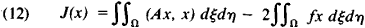where Ω is a region bounded by curve Г.

Equation (11) in this case is the Euler equation for functional (12).

The reduction of problem (11) to problem (12) is possible, for example, if A is a self-adjoint and positive-definite operator. The Laplace operator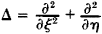satisfies these requirements. The connection between the problems of partial differential equations and variational problems is of great practical value. In particular, it permits the determination of the validity of various existence and uniqueness theorems and has played an important role in the crystallization of the concept of the generalized solution. This reduction is very important also in computational mathematics, since it permits the use of the direct methods of the calculus of variations.

In the enumeration of the primary branches of modern calculus of variations, it is impossible not to point out the universal problems of the calculus of variations, whose solutions require qualitative methods. The desired solution of a variational problem satisfies some complex nonlinear equation and boundary conditions. It is natural to pose the question of how many solutions this problem permits. An example of such a problem is that of the number of geodesies that can be constructed between two points on a given surface. A similar-type problem is already considered to be part of the qualitative theory of differential equations and topology. The last circumstance is very characteristic. The methods that are specific for related disciplines, topology, functional analysis, and so forth have increasingly begun to be applied in the calculus of variations. In their turn, the ideas of the calculus of variations are penetrating into all the new fields of mathematics and the boundary between the calculus of variations and related mathematical fields has become difficult to define.

### REFERENCES

Lavrent’ev, M. A., and L. A. Liusternik. Kurs variatsionnogo ischisleniia, 2nd ed. Moscow-Leningrad, 1950.
Bliss, G. A. Lektsii po variatsionnomu ischisleniiu. Moscow, 1950. (Translated from English.)
Mikhlin, S. G. Variatsionnye metody v matematicheskoi fizike. Moscow, 1957.
Smirnov, V. I. Kurs vysshei matematiki, 5th ed. vol. 4. Moscow, 1958.
Gel’fand, I. M., and S. V. Fomin. Variatsionnoe ischislenie. Moscow, 1961.
Matematicheskaia teoriia optimal’nykh protsessov. Moscow, 1969.

N. N. MOISEEV

## calculus of variations

[′kal·kyə·ləs əv ‚ver·ē′ā·shənz]
(mathematics)
The study of problems concerning maximizing or minimizing a given definite integral relative to the dependent variables of the integrand function.
References in periodicals archive ?
A comparison in the theory of calculus of variations on time scales with an application to the Ramsey model.
In Section 5 we specify these new transformations to the calculus of variations setting (with and without shift in the state variable) and compare these new results with the known transformations in the literature.
Fomin, Calculus of Variations, Prentice-Hall, Englewood Cliffs, NJ, 1963.
After a few remarks on the historical development, the second part provides an introduction to the calculus of variations and the relationship between integral equations and applications of the calculus of variations.
The authors have organized the main body of their text in thirteen chapters devoted to the calculus of variations and optimal control, fractional calculus, fractional variational problems, and a variety of other related subjects.
Specific applied mathematics topics covered include intergro-differential equations, the use of the Fourier transformation on time-series data and calculus of variations.
The first edition was published in 2003; among the changes in the second are new chapters on the Volterra equations and the calculus of variations.
A primer on the calculus of variations and optimal control theory.
A sampling of topics includes: double refraction, electromagnetism, liquid crystals, the calculus of variations, Maier-Saupe theory, static continuum theory, LCD chemistry, engineering, the active matrix, the transistor and integrated circuit, and new technologies and products.
Paul Malliavin developed the stochastic calculus of variations that bears his name in 1976 primarily to establish the regularity of the probability distribution of functionals of an underlying Gaussian process.
Specialists in optimal control theory and the calculus of variations working on problems on unbounded domains attended the workshop present papers more or less reflecting the talks they delivered at the workshop.

Site: Follow: Share:
Open / Close